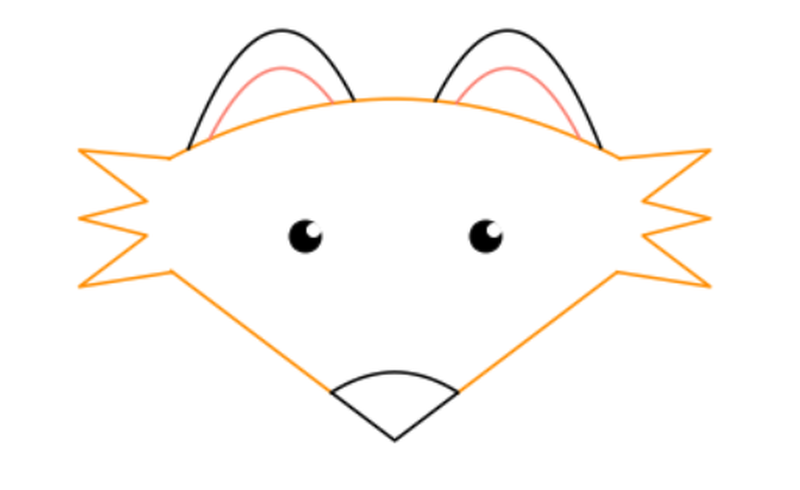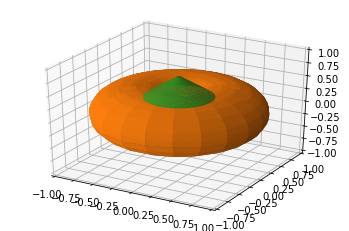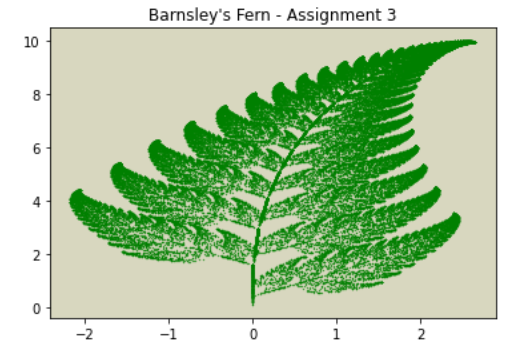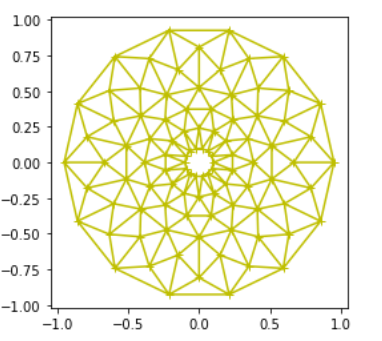As part of the University of North Carolina BIOL222 class, Dr. Catherine Kehl asked her students to “use matplotlib.pyplot to make art.” BIOL222 is Introduction to Programming, aimed at students with no programming background. The emphasis is on practical, hands-on active learning.

The students completed the assignment with festive enthusiasm around Halloween. Here are some great examples:

Harris Davis showed an affinity for pumpkins, opting to go 3D!# get library for 3d plotting

from mpl_toolkits.mplot3d import Axes3D

# make a pumpkin :)

rho = np.linspace(0, 3*np.pi,32)
theta, phi = np.meshgrid(rho, rho)
r, R = .5, .5
X = (R + r * np.cos(phi)) * np.cos(theta)
Y = (R + r * np.cos(phi)) * np.sin(theta)
Z = r * np.sin(phi)

# make the stem

theta1 = np.linspace(0,2*np.pi,90)
r1 = np.linspace(0,3,50)
T1, R1 = np.meshgrid(theta1, r1)
X1 = R1 * .5*np.sin(T1)
Y1 = R1 * .5*np.cos(T1)
Z1 = -(np.sqrt(X1**2 + Y1**2) - .7)
Z1[Z1 < .3] = np.nan
Z1[Z1 > .7] = np.nan

# Display the pumpkin & stem

fig = plt.figure()
ax = fig.gca(projection = '3d')
ax.set_xlim3d(-1, 1)
ax.set_ylim3d(-1, 1)
ax.set_zlim3d(-1, 1)
ax.plot_surface(X, Y, Z, color = 'tab:orange', rstride = 1, cstride = 1)
ax.plot_surface(X1, Y1, Z1, color = 'tab:green', rstride = 1, cstride = 1)
plt.show()


Bryce Desantis stuck to the biological theme and demonstrated fractal art.import numpy as np
import matplotlib.pyplot as plt

#Barnsley's Fern - Fractal; en.wikipedia.org/wiki/Barnsley_…

#functions for each part of fern:

#stem

def stem(x,y):
return (0, 0.16*y)
#smaller leaflets

def smallLeaf(x,y):
return (0.85*x + 0.04*y, -0.04*x + 0.85*y + 1.6)
#large left leaflets

def leftLarge(x,y):
return (0.2*x - 0.26*y, 0.23*x + 0.22*y + 1.6)
#large right leftlets

def rightLarge(x,y):
return (-0.15*x + 0.28*y, 0.26*x + 0.24*y + 0.44)
componentFunctions = [stem, smallLeaf, leftLarge, rightLarge]

# number of data points and frequencies for parts of fern generated:

#lists with all 75000 datapoints

datapoints = 75000
x, y = 0, 0
datapointsX = []
datapointsY = []
#For 75,000 datapoints

for n in range(datapoints):
FrequencyFunction = np.random.choice(componentFunctions, p=[0.01, 0.85, 0.07, 0.07])
x, y = FrequencyFunction(x,y)
datapointsX.append(x)
datapointsY.append(y)

plt.scatter(datapointsX,datapointsY,s=0.1, color='g')
#Title of Figure

plt.title("Barnsley's Fern - Assignment 3")
#Changing background color

ax = plt.axes()
ax.set_facecolor("#d8d7bf")


Grace Bell got a little trippy with this rotationally semetric art. It's pretty cool how she captured mouse events. It reminds us of a flower. What do you see?import matplotlib.pyplot as plt
from matplotlib.tri import Triangulation
from matplotlib.patches import Polygon
import numpy as np

#I found this sample code online and manipulated it to make the art piece!

#was interested in because it combined what we used for functions as well as what we used for plotting with (x,y)

def update_polygon(tri):
if tri == -1:
points = [0, 0, 0]
else:
points = triang.triangles[tri]
xs = triang.x[points]
ys = triang.y[points]
polygon.set_xy(np.column_stack([xs, ys]))

def on_mouse_move(event):
if event.inaxes is None:
tri = -1
else:
tri = trifinder(event.xdata, event.ydata)
update_polygon(tri)
ax.set_title(f'In triangle {tri}')
event.canvas.draw()
#this is the info that creates the angles

n_angles = 14
min_radius = 0.1 #the radius of the middle circle can move with this variable

angles = np.linspace(0, 2 * np.pi, n_angles, endpoint=False)
angles = np.repeat(angles[..., np.newaxis], n_radii, axis=1)
angles[:, 1::2] += np.pi / n_angles
triang = Triangulation(x, y)
y[triang.triangles].mean(axis=1))

trifinder = triang.get_trifinder()

fig, ax = plt.subplots(subplot_kw={'aspect': 'equal'})
ax.triplot(triang, 'y+-') #made the color of the plot yellow and there are "+" for the data points but you can't really see them because of the lines crossing

polygon = Polygon([[0, 0], [0, 0]], facecolor='y')
update_polygon(-1)
fig.canvas.mpl_connect('motion_notify_event', on_mouse_move)
plt.show()


As a bonus, did you like that fox in the banner? That was created (and well documented) by Emily Foster!

import numpy as np
import matplotlib.pyplot as plt

plt.axis('off')

#outer ears

xearL = np.arange(-45.8,-9,0.1)
yearL = -0.08*(xearL*xearL) -4*xearL + 70

xearR = np.arange(9,45.8,0.1)
yearR = -0.08*(xearR*xearR) + 4*xearR + 70

plt.plot(xearL, yearL, 'black')
plt.plot(xearR, yearR, 'black')

#inner ears

xinL = np.arange(-41.1,-13.7,0.1)
yinL = -0.08*(xinL*xinL) -4*xinL + 59

xinR = np.arange(13.7,41.1,0.1)
yinR = -0.08*(xinR*xinR) + 4*xinR + 59

plt.plot(xinL, yinL, 'salmon')
plt.plot(xinR, yinR, 'salmon')

# bottom of face

xfaceL = np.arange(-49.6,-14,0.1)
xfaceR = np.arange(14,49.3,0.1)
xfaceM = np.arange(-14,14,0.1)

plt.plot(xfaceL, abs(xfaceL), 'darkorange')
plt.plot(xfaceR, abs(xfaceR), 'darkorange')
plt.plot(xfaceM, abs(xfaceM), 'black')

#nose

xnose = np.arange(-14,14,0.1)
ynose = -0.03*(xnose*xnose) + 20

plt.plot(xnose, ynose, 'black')

#whiskers

xwhiskR = [50, 70, 55, 70, 55, 70, 49.3]
xwhiskL = [-50, -70, -55, -70, -55, -70, -49.3]
ywhisk = [82.6, 85, 70, 65, 60, 45, 49.3]

plt.plot(xwhiskR, ywhisk, 'darkorange')
plt.plot(xwhiskL, ywhisk, 'darkorange')

#eyes

plt.plot(20,60, color = 'black', marker = 'o', markersize = 15)
plt.plot(-20,60,color = 'black', marker = 'o', markersize = 15)

plt.plot(22,62, color = 'white', marker = 'o', markersize = 6)
plt.plot(-18,62,color = 'white', marker = 'o', markersize = 6)


We look forward to seeing these students continue in their plotting and scientific adventures!